# RS Aggarwal Solutions for Class 8 Maths Chapter 22 - Introduction to Coordinate Geometry

RS Aggarwal Solutions For Class 8 Maths Chapter 22 Introduction to Coordinate Geometry are provided here. You can download the pdf of RS Aggarwal Solutions for Class 8 Maths Chapter 22 Introduction to Coordinate Geometry from the given links. Class 8 is an important phase of a student’s life. It is critical to thoroughly understand the concepts taught in Class 8 as they are continued in Class 9 and 10, and also build a foundation. Here we will learn about coordinate geometry, Cartesian plane.

RS Aggarwal Solutions helps students to get a good score in the examinations, while also providing extensive knowledge about the subject, as Class 8 is a critical stage in their academic career. Therefore, we at BYJU’S provide answers to all questions uniquely and briefly.

## Download pdf of RS Aggarwal Solutions For Class 8 Maths Chapter 22 Introduction To Coordinate Geometry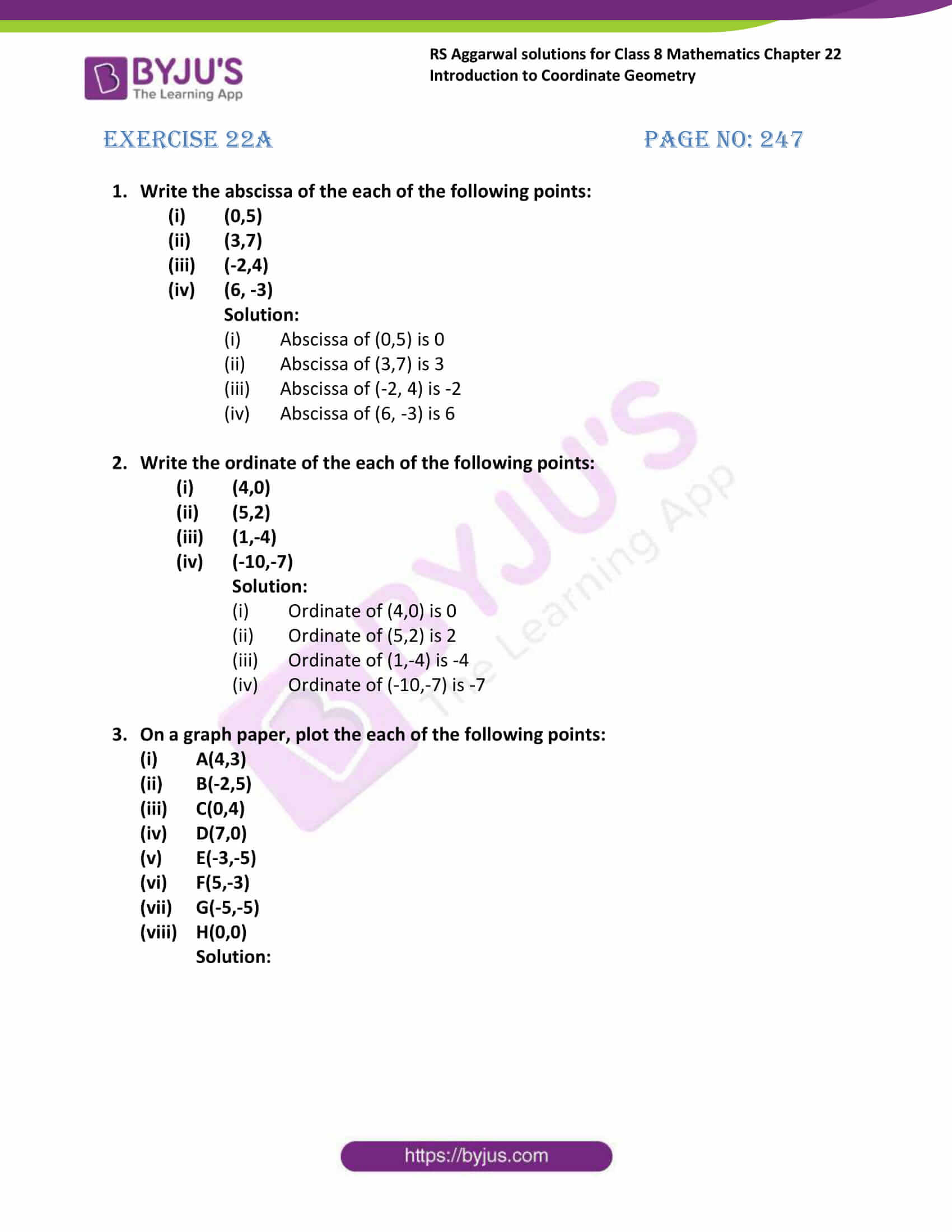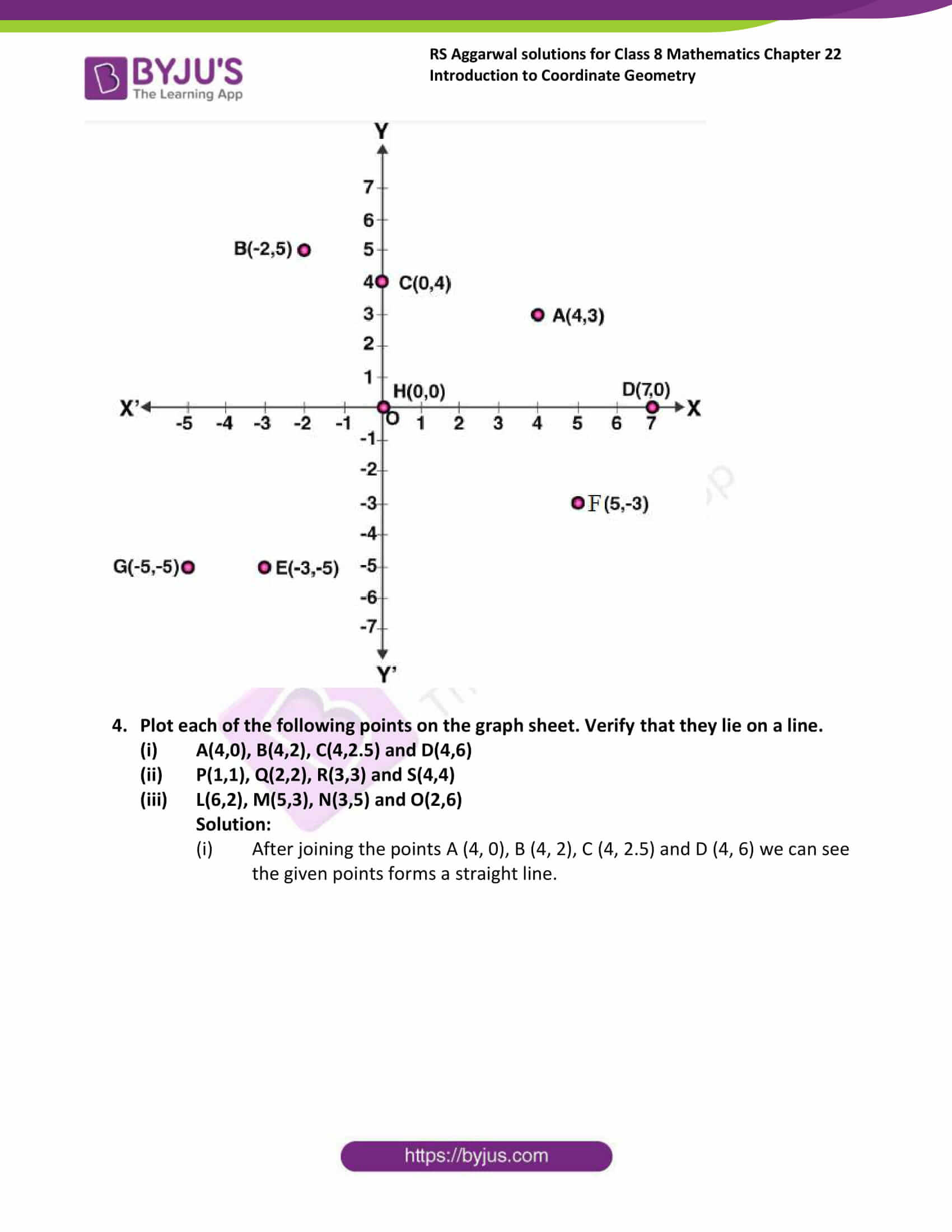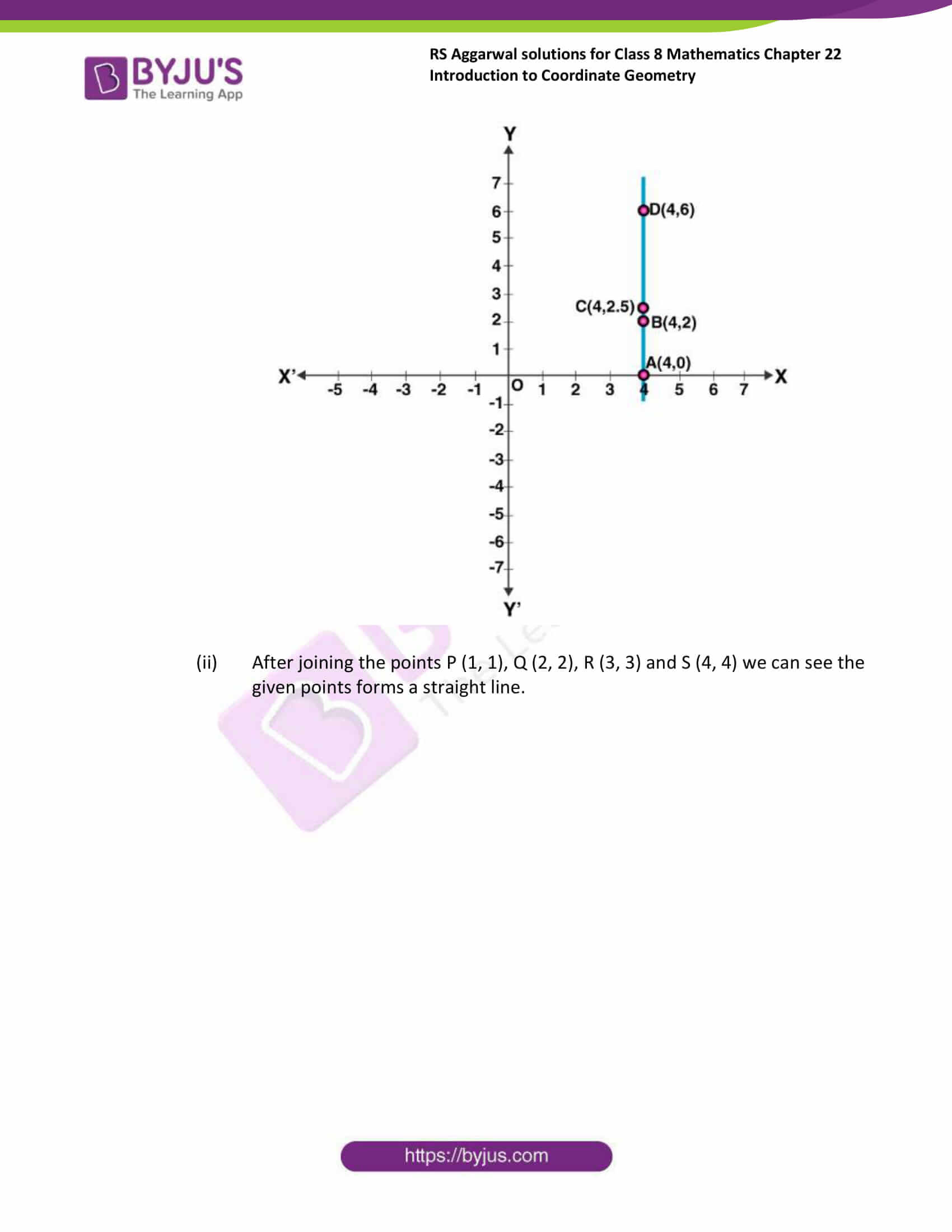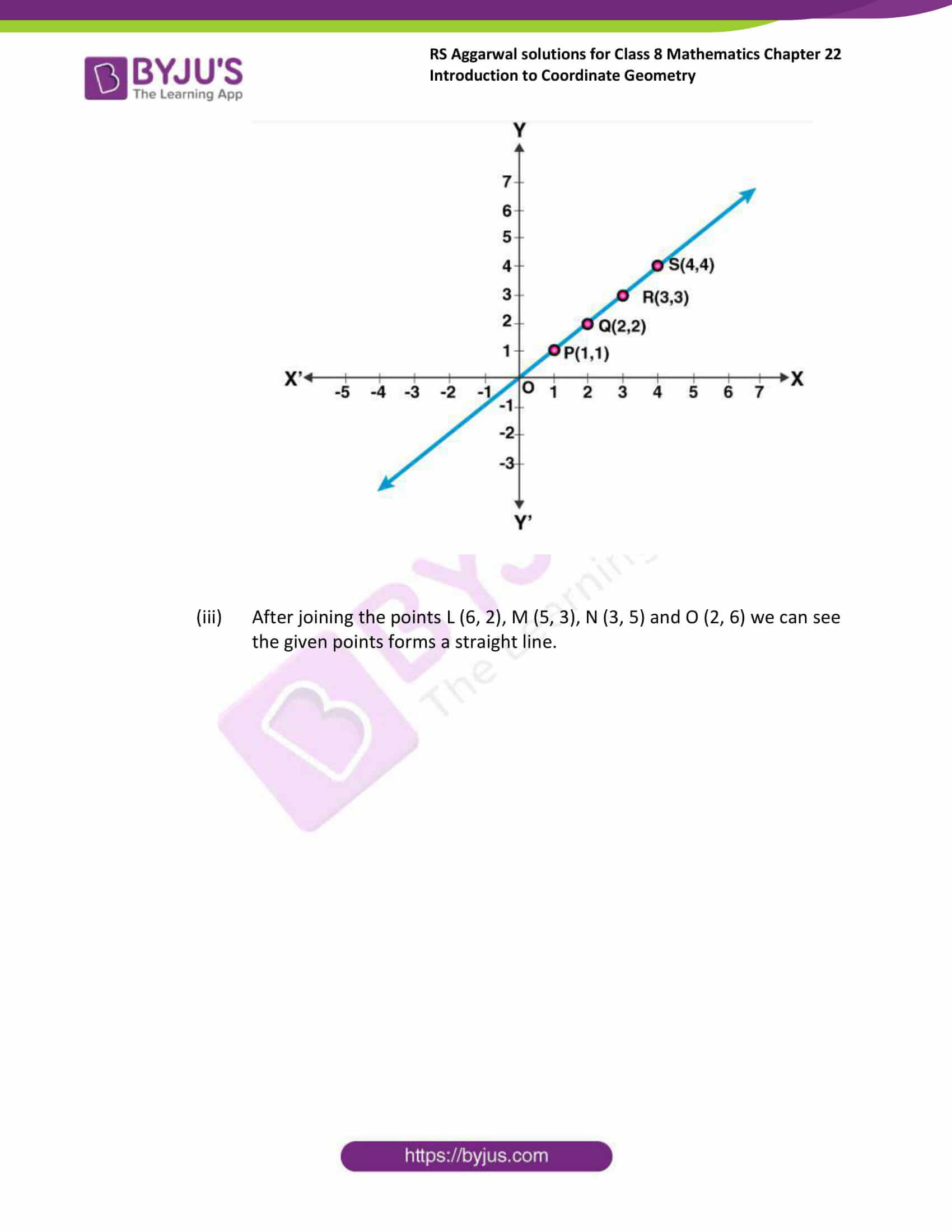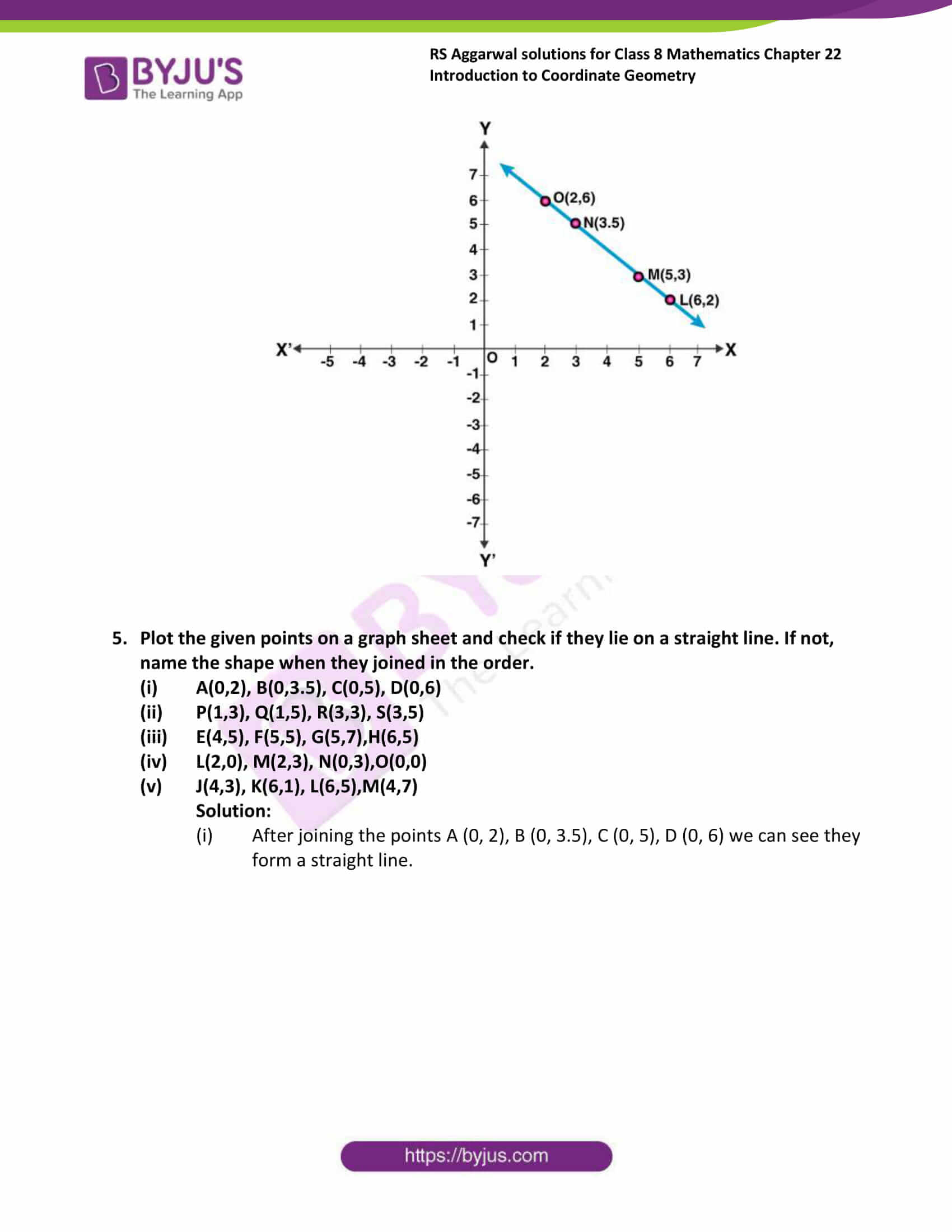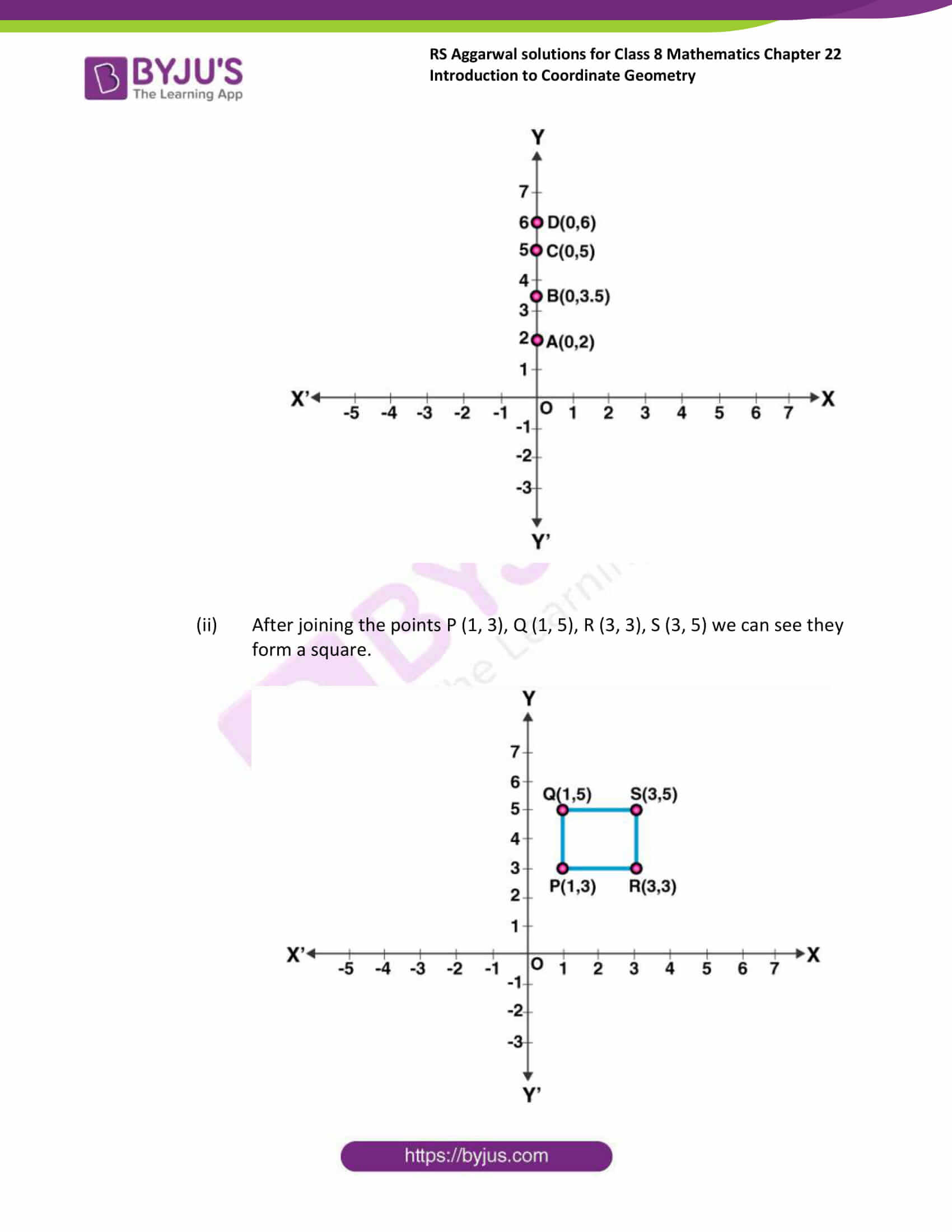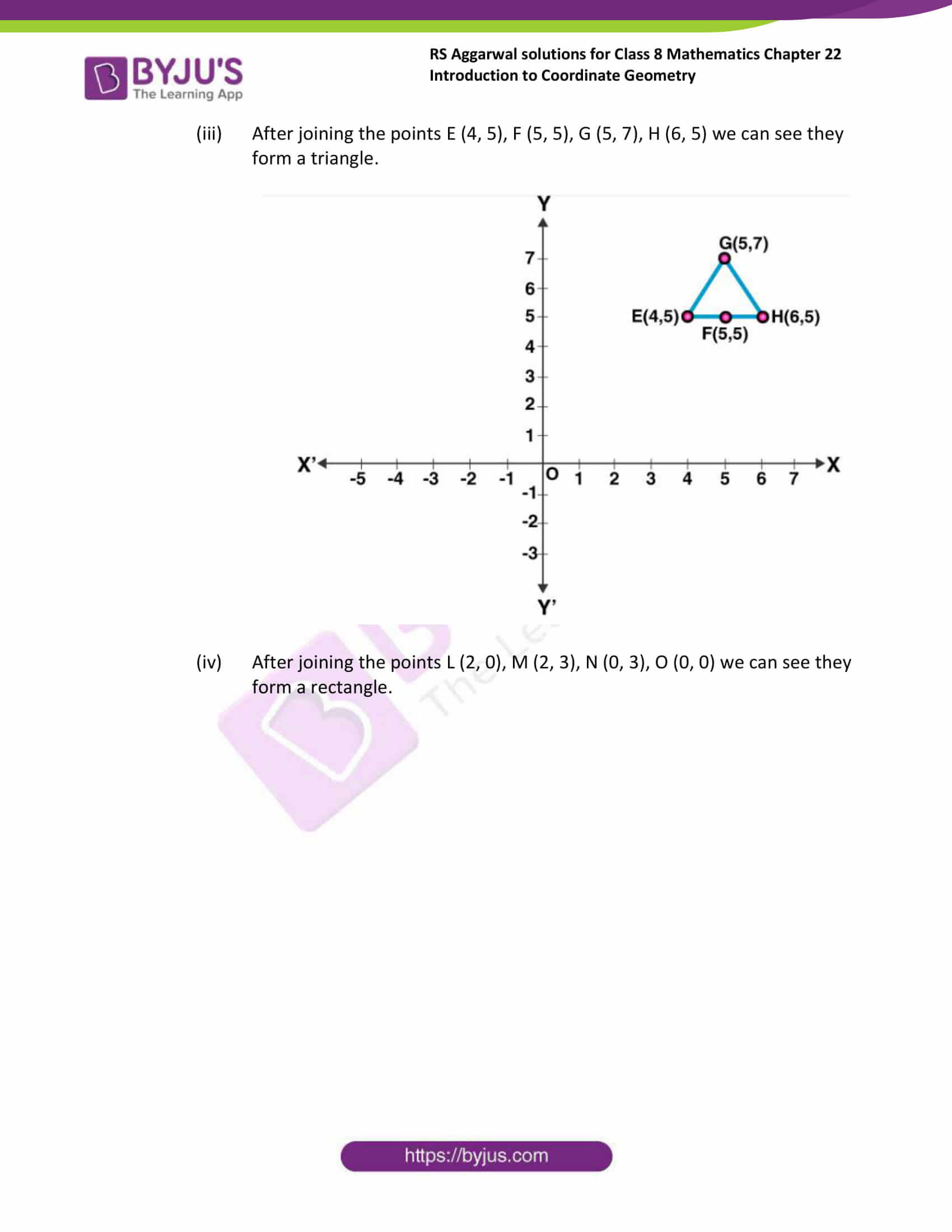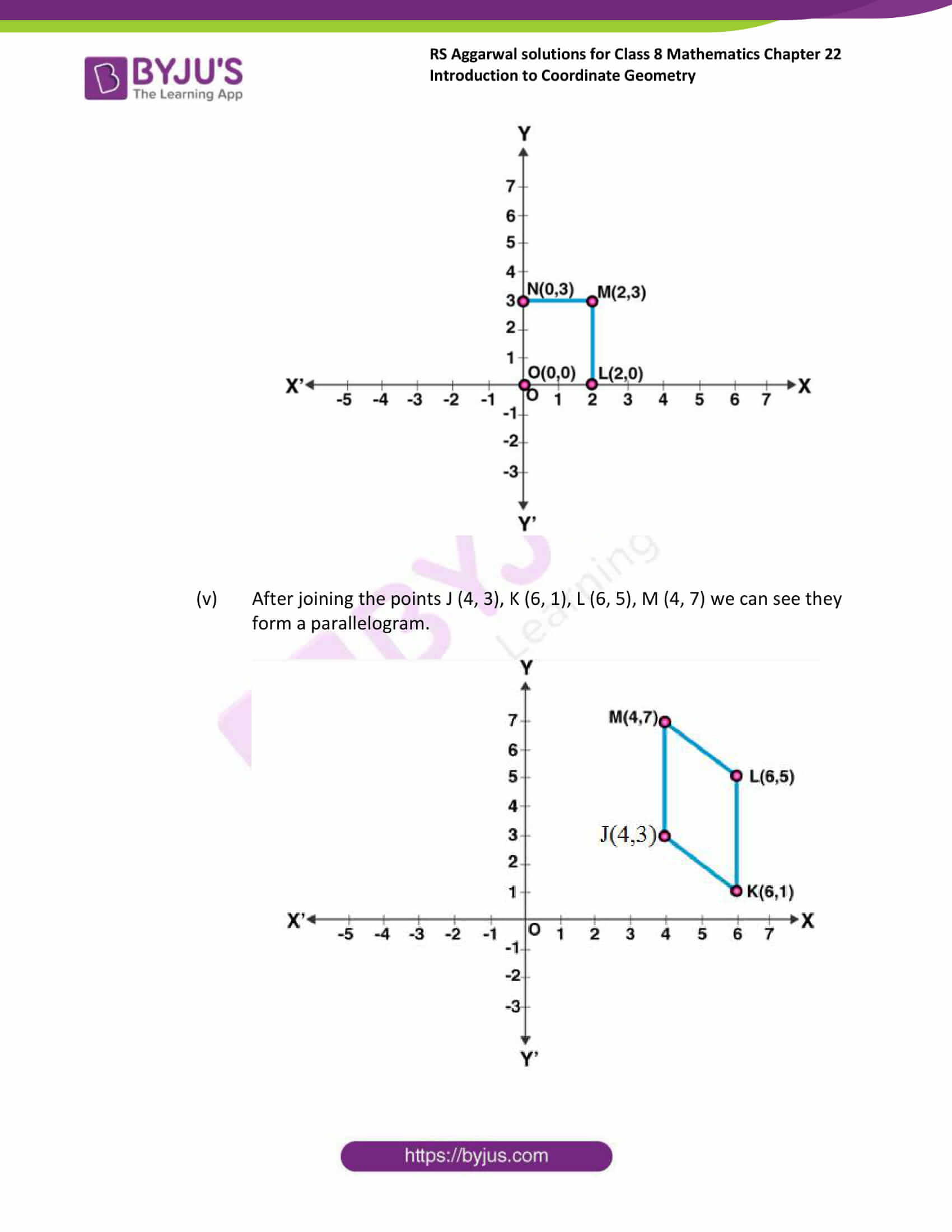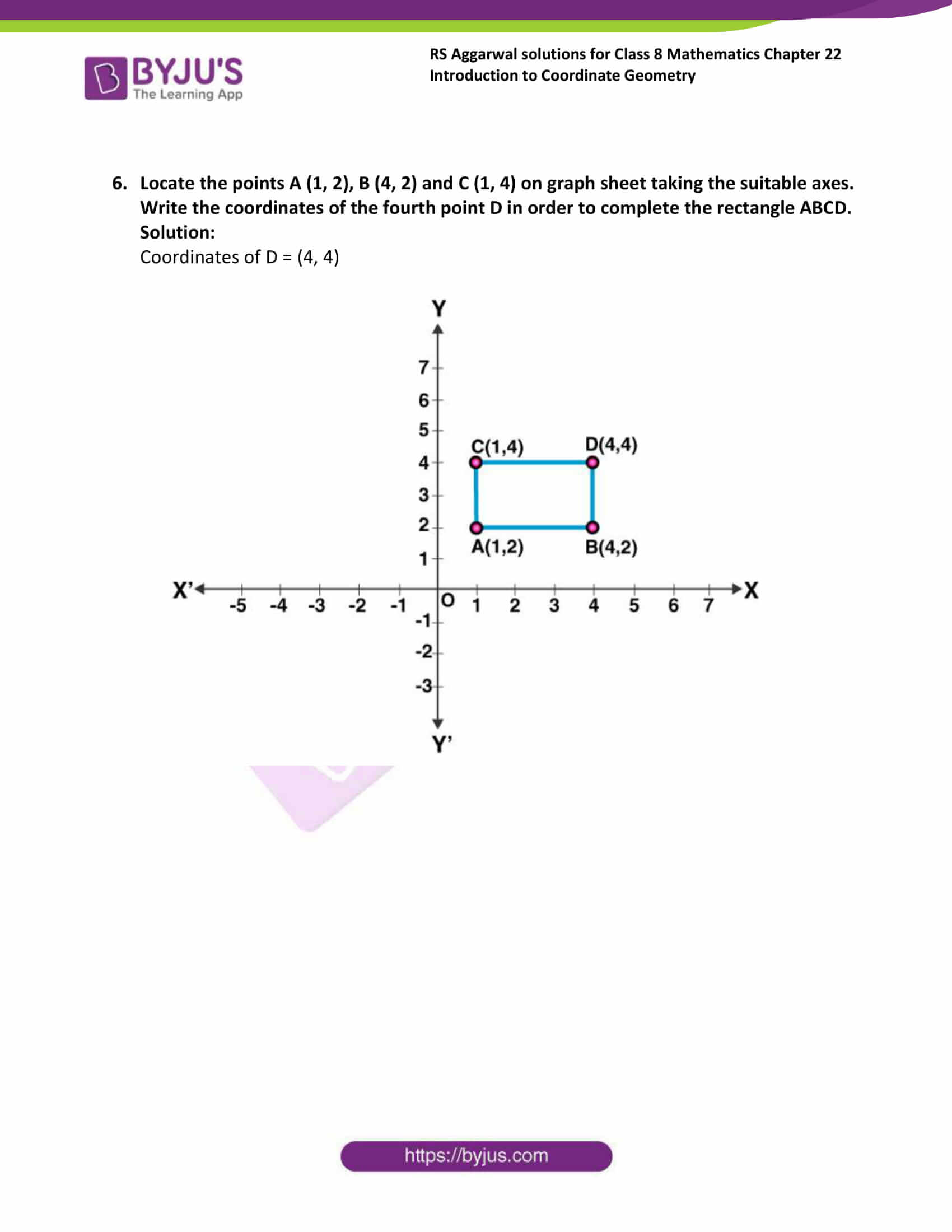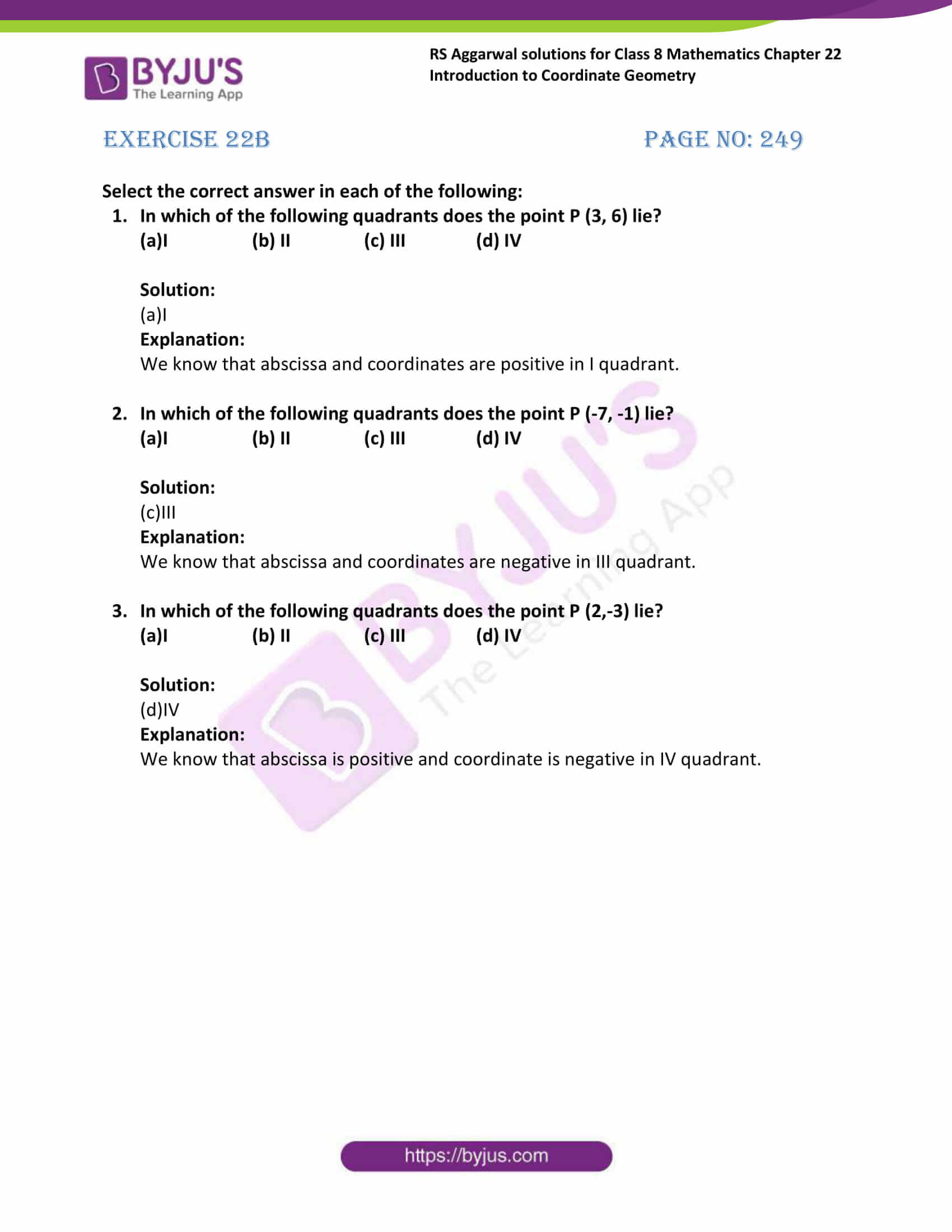### Access Answers to Maths RS Aggarwal Solutions for Class 8 Chapter 22 – Introduction To Coordinate Geometry

Exercise 22A Page No: 247

1. Write the abscissa of the each of the following points:

(i) (0,5)

(ii) (3,7)

(iii) (-2,4)

(iv) (6, -3)

Solution:

(i) Abscissa of (0,5) is 0

(ii) Abscissa of (3,7) is 3

(iii) Abscissa of (-2, 4) is -2

(iv) Abscissa of (6, -3) is 6

2. Write the ordinate of the each of the following points:

(i) (4,0)

(ii) (5,2)

(iii) (1,-4)

(iv) (-10,-7)

Solution:

(i) Ordinate of (4,0) is 0

(ii) Ordinate of (5,2) is 2

(iii) Ordinate of (1,-4) is -4

(iv) Ordinate of (-10,-7) is -7

3. On a graph paper, plot the each of the following points:

(i) A(4,3)

(ii) B(-2,5)

(iii) C(0,4)

(iv) D(7,0)

(v) E(-3,-5)

(vi) F(5,-3)

(vii) G(-5,-5)

(viii) H(0,0)

Solution: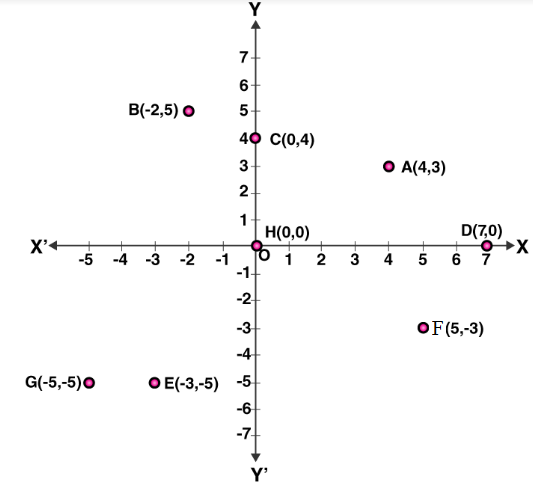4. Plot each of the following points on the graph sheet. Verify that they lie on a line.

(i) A(4,0), B(4,2), C(4,2.5) and D(4,6)

(ii) P(1,1), Q(2,2), R(3,3) and S(4,4)

(iii) L(6,2), M(5,3), N(3,5) and O(2,6)

Solution:

(i) After joining the points A (4, 0), B (4, 2), C (4, 2.5) and D (4, 6) we can see the given points forms a straight line.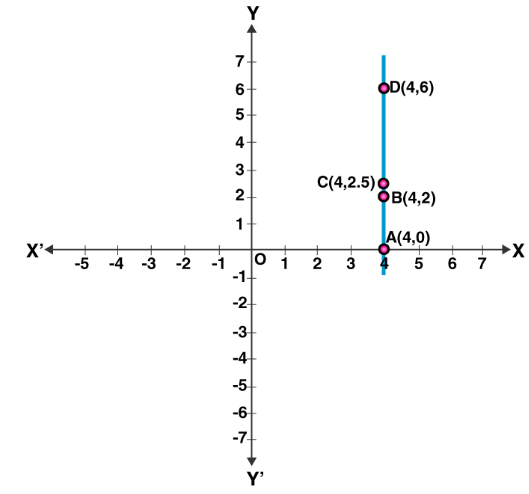(ii) After joining the points P (1, 1), Q (2, 2), R (3, 3) and S (4, 4) we can see the given points forms a straight line.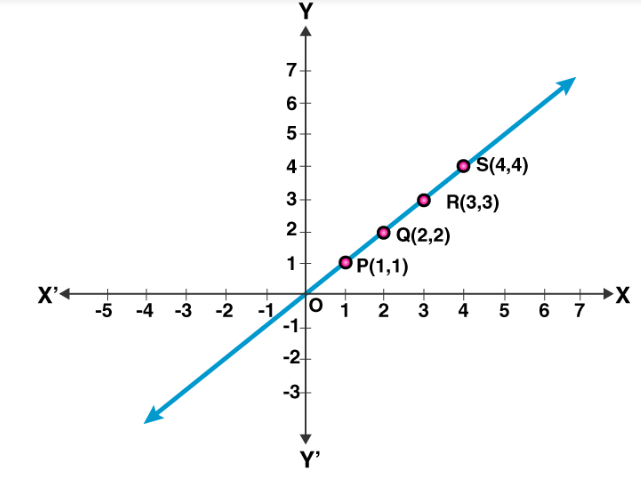(iii) After joining the points L (6, 2), M (5, 3), N (3, 5) and O (2, 6) we can see the given points forms a straight line.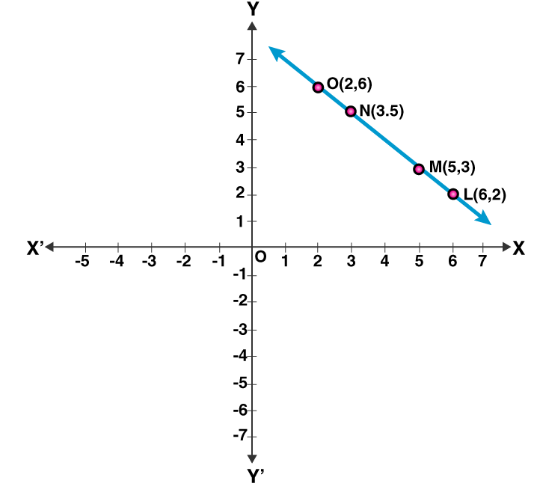5. Plot the given points on a graph sheet and check if they lie on a straight line. If not, name the shape when they joined in the order.

(i) A(0,2), B(0,3.5), C(0,5), D(0,6)

(ii) P(1,3), Q(1,5), R(3,3), S(3,5)

(iii) E(4,5), F(5,5), G(5,7),H(6,5)

(iv) L(2,0), M(2,3), N(0,3),O(0,0)

(v) J(4,3), K(6,1), L(6,5),M(4,7)

Solution:

(i) After joining the points A (0, 2), B (0, 3.5), C (0, 5), D (0, 6) we can see they form a straight line.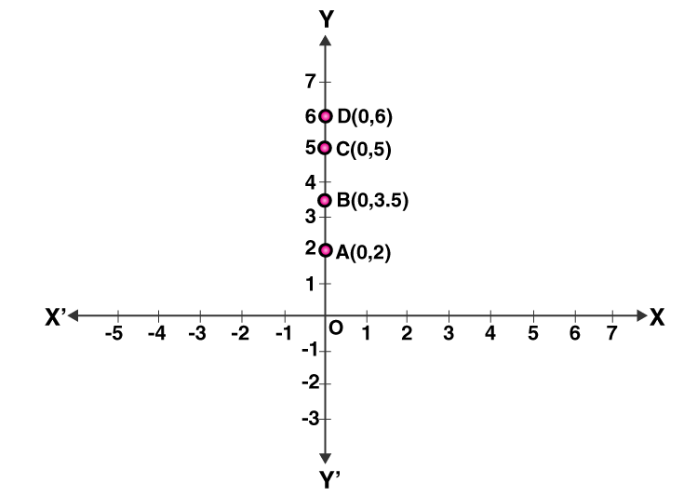(ii) After joining the points P (1, 3), Q (1, 5), R (3, 3), S (3, 5) we can see they form a square.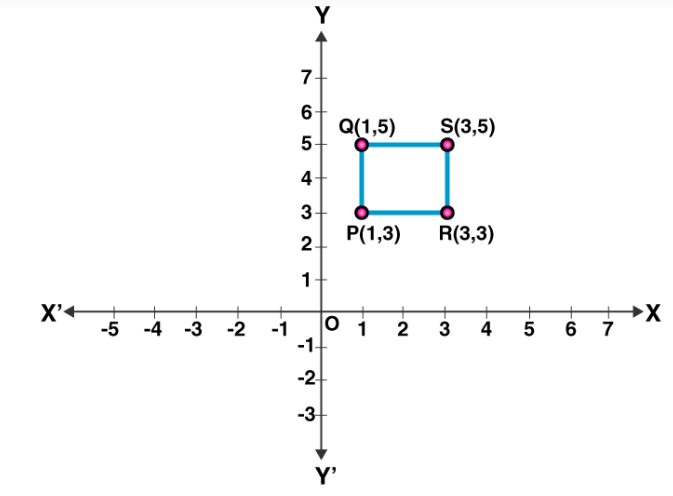(iii) After joining the points E (4, 5), F (5, 5), G (5, 7), H (6, 5) we can see they form a triangle.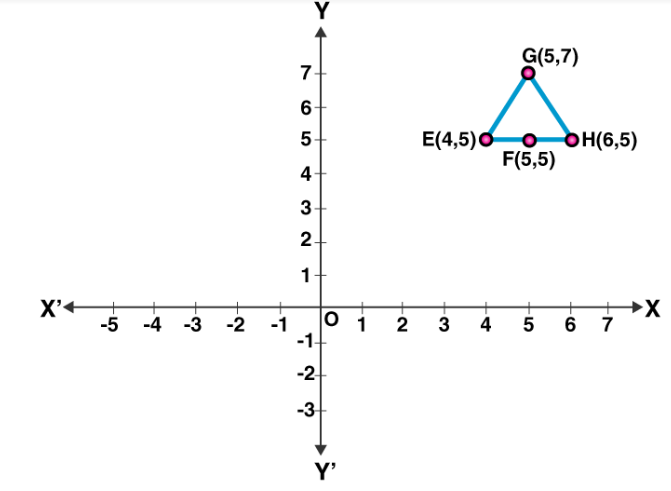(iv) After joining the points L (2, 0), M (2, 3), N (0, 3), O (0, 0) we can see they form a rectangle.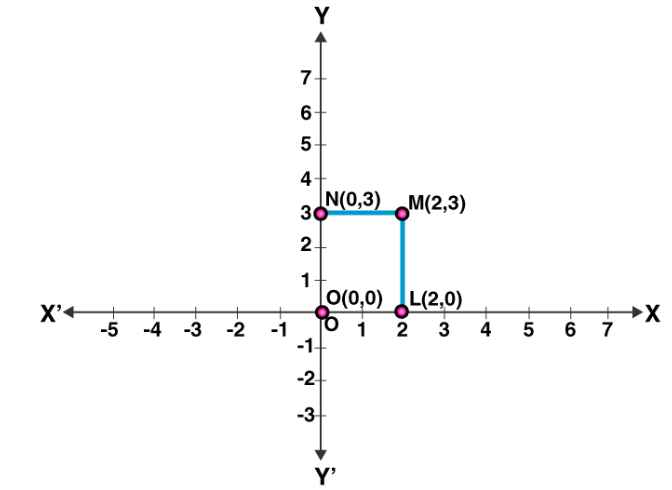(v) After joining the points J (4, 3), K (6, 1), L (6, 5), M (4, 7) we can see they form a parallelogram.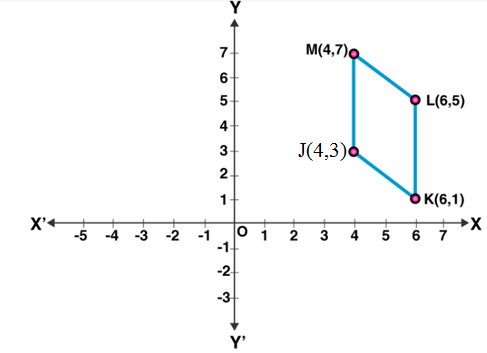6. Locate the points A (1, 2), B (4, 2) and C (1, 4) on graph sheet taking the suitable axes. Write the coordinates of the fourth point D in order to complete the rectangle ABCD.

Solution:

Coordinates of D = (4, 4)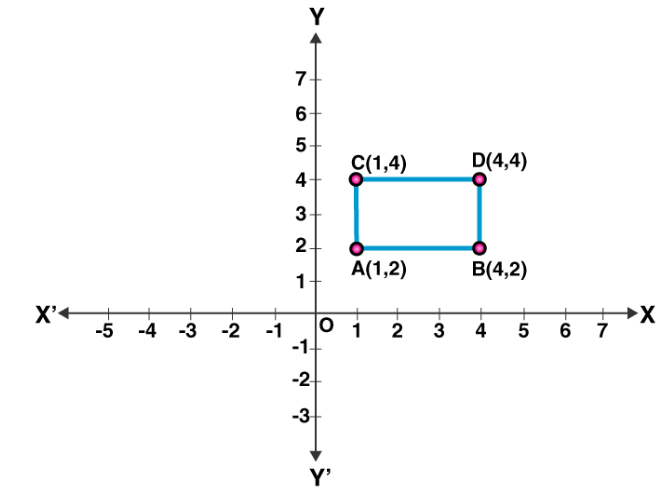Exercise 22B Page No: 249

Select the correct answer in each of the following:

1. In which of the following quadrants does the point P (3, 6) lie?

(a)I (b) II (c) III (d) IV

Solution:

(a)I

Explanation:

We know that abscissa and coordinates are positive in I quadrant.

2. In which of the following quadrants does the point P (-7, -1) lie?

(a)I (b) II (c) III (d) IV

Solution:

(c) III

Explanation:

We know that abscissa and coordinates are negative in III quadrant.

3. In which of the following quadrants does the point P (2,-3) lie?

(a)I (b) II (c) III (d) IV

Solution:

(d)IV

Explanation:

We know that abscissa is positive and coordinate is negative in IV quadrant.

## RS Aggarwal Solutions for Class 8 Maths Chapter 22 – Introduction To Coordinate Geometry

Chapter 22, Introduction to Coordinate Geometry, contains 2 Exercises. RS Aggarwal Solutions given here contains the answers to all the questions present in these exercises. Let us have a look at some of the concepts that are being discussed in this Chapter.

Coordinate axis
Cartesian plane
Definition of order pair
Meaning of coordinates of a point